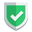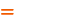我们相信：世界是美好的，你是我也是。平行空间的世界里面，不同版本的生活也在继续...

## 准备数据

``````import jieba
import pandas as pd
from sklearn.feature_extraction.text import CountVectorizer
from sklearn.naive_bayes import MultinomialNB``````

``````stopwords = []
with open('./data/stopwords.txt', 'r', encoding='utf-8') as f:
for line in lines:
stopwords.append(line.strip())
stopwords = list(set(stopwords))  # 去重``````

## 分词

``````data = pd.read_csv("./data/评价.csv", encoding="gbk")
_text = []
for c in data["内容"]:
_seg = jieba.cut(c, cut_all=False)
_text.append(','.join(_seg))``````

## 统计词频

``````con = CountVectorizer(stop_words=stopwords)
X = con.fit_transform(_text)``````

## 训练集和测试集

``````_x = X.toarray()
_y = data['评价'].values``````

### 训练集分割方案一

``````X_train = _x[:20, :]
y_train = _y[:20]
# print(X_train, y_train)
X_test = _x[20:, :]
y_test = _y[20:]
# print(X_test, y_test)``````

### 训练集分割方案二

``````from sklearn.model_selection import train_test_split
X_train, X_test, y_train, y_test = train_test_split(_x, _y, test_size=0.2)``````

### 训练集分割方案三【推荐】

``````from sklearn.model_selection import train_test_split
X_train, X_test, y_train, y_test = train_test_split(
_x, _y, test_size=0.2, random_state=1, shuffle=False)``````
• `random_state`，这个取啥整数值都可以，只是为了保持一致。
• `shuffle`，默认为`true`打乱，改成`false`就是不打乱。

## 朴素贝叶斯预测

• 先使用`.fit()`来做训练，第一个输入是把单词变成0,1的数组，第二个输入是这些数组元素对应的结果（真实的结论）。
• 使用`.predict()`来做预测，输入是和上面的第一个输入同样处理的数组，输出是预测的结果，格式类似上面的第二个输入。
``````mb = MultinomialNB(alpha=1)
mb.fit(X_train, y_train)
y_predict = mb.predict(X_test)``````

``````print('真实值：', y_test)
print('预测值：', y_predict)
print(mb.score(X_test, y_test))``````

``````真实值： ['好评' '好评' '差评' '差评' '好评' '好评']

1.0``````

• 使用更多更准确的训练集。
• 使用`stop_words`去掉训练集中无关的词。

## 综述如果本文对您有帮助，或者节约了您的时间，欢迎打赏瓶饮料，建立下友谊关系。本博客不欢迎：各种镜像采集行为。请尊重原创文章内容，转载请保留作者链接。【福利】 腾讯云最新爆款活动！1核2G云服务器首年50元！【源码】本文代码片段及相关软件，请点此获取更多信息【绝密】秘籍文章入口，仅传授于有缘之人python    sklearn